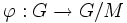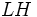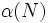# Hall retract implies order-conjugate

This article describes a fact or result that is not basic but it still well-established and standard. The fact may involve terms that are themselves non-basic
View other semi-basic facts in group theory
VIEW FACTS USING THIS: directly | directly or indirectly, upto two steps | directly or indirectly, upto three steps|
This article gives the statement and possibly, proof, of an implication relation between two subgroup properties. That is, it states that every subgroup satisfying the first subgroup property (i.e., Hall retract) must also satisfy the second subgroup property (i.e., order-conjugate subgroup)
View all subgroup property implications | View all subgroup property non-implications
This article states and (possibly) proves a fact that involves two finite groups of relatively prime order, requiring the additional datum that at least one of them is solvable. Due to the Feit-Thompson theorem, we know that for two finite groups of relatively prime orders, one of them is solvable. Hence, the additional datum of solvability can be dropped. However, the proof of the Feit-Thompson theorem is considered heavy machinery.
View more such facts

## Name

This result is often stated as the conjugacy part of the Schur-Zassenhaus theorem.

## Statement

Suppose$G$ is a finite group and$H$ is a Hall retract of$G$. In other words,$H$ is a Hall subgroup of$G$ that is also a retract: there exists a normal complement$N$ to$H$ in$G$. Note that$N$ is thus a normal Hall subgroup. Assume, further, that either$N$ or$H$ is a solvable group.

Then, if there is any subgroup$K$ of$G$ of the same order as$H$,$H$ and$K$ are conjugate subgroups.

Note that the assumption that either$N$ or$H$ is a solvable group is superfluous because, as a corollary of the odd-order theorem, given two groups of coprime order, one of them is solvable.

## Proof

We reduce the problem to basic cases by induction on the order.

Given: A finite group$G$. A normal$\pi$-Hall subgroup$N$ of$G$, and two$\pi'$Hall subgroups$H,K$ of$G$.

To prove:$H$ is conjugate to$K$.

Proof:

1. Reduction to the case that$N$ is minimal normal in$G$: Suppose$M$ is a nontrivial normal subgroup of$G$ properly contained in$N$. Let$\varphi:G \to G/M$ be the quotient map.$\varphi(N)$ is normal$\pi$-Hall in$G/N$, and$\varphi(H), \varphi(K)$ are$\pi'$-Hall subgroups. By the inductive hypothesis,$\varphi(H)$ and$\varphi(K)$ are conjugate in$G/N$. Suppose$g \in G$ is such that$\varphi(g)$ conjugates$\varphi(H)$ to$\varphi(K)$. Then,$g$ conjugates$H$ to some$\pi'$-Hall subgroup inside$\varphi^{-1}(\varphi(K)) = KM$. Thus, it suffices to show that any$\pi'$-Hall subgroup of$KM$ is conjugate to$K$. This again follows by the inductive hypothesis on the order, since$M$ is normal$\pi$-Hall in$KM$.
2. Reduction to the case that$O_{\pi'}(G)$ is trivial; in other words,$G$ has no nontrivial normal$\pi'$-subgroups: Suppose$L$ is a nontrivial normal$\pi'$-subgroup of$G$. Then, by the product formula,$LH$ and$LK$ are$\pi'$-subgroups, and since$H,K$ are Hall, this forces$L \le H$ and$L \le K$. Consider the quotient map$\alpha:G \to G/L$. Under this quotient map,$\alpha(N)$ is a normal$\pi$-Hall subgroup, and$\alpha(H), \alpha(K)$ are$\pi'$-Hall. The inductive hypothesis yields that$\alpha(H)$ and$\alpha(K)$ are conjugate in$G/L$. Since$H$ and$K$ both contain the kernel of$\alpha$, this yields that$H$ and$K$ are conjugate in$G$.
3. Resolution of the case where$H$ is solvable: PLACEHOLDER FOR INFORMATION TO BE FILLED IN: [SHOW MORE]
4. Resolution of the case where$N$ is solvable: PLACEHOLDER FOR INFORMATION TO BE FILLED IN: [SHOW MORE]